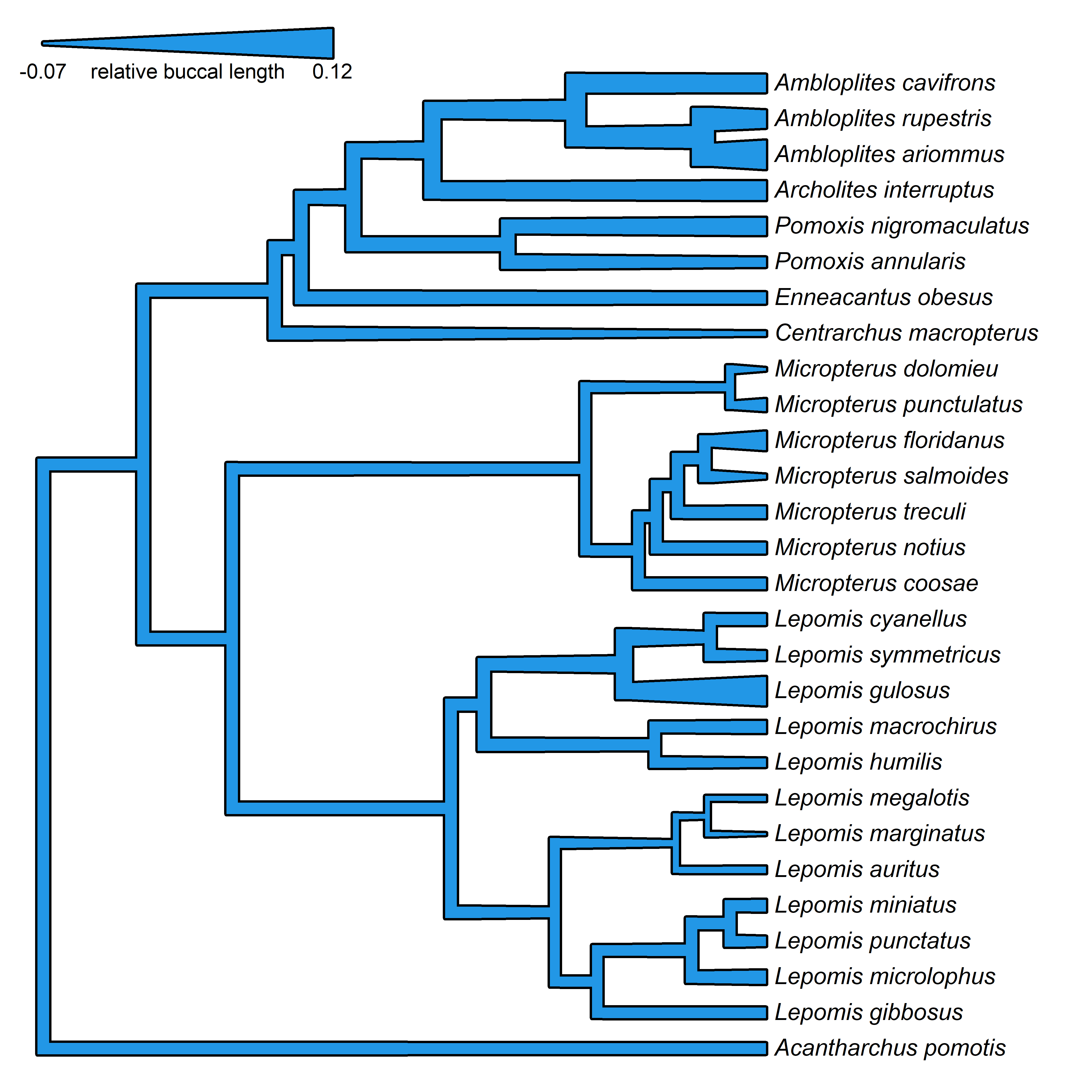## Monday, July 20, 2020

### Mapping a continuous trait onto the branches of a phylogeny using variable edge widths: Part IV

A couple of days ago I posted some code showing how to plot a right- (or left-) facing phylogram with polygons for branches.

As of this afternoon, I have just pushed these new functions to the phytools GitHub page. This update can be obtained by downloading & installing phytools directly from GitHub using devtools.

``````library(devtools)
install_github("liamrevell/phytools")
``````

Beyond what I showed yesterday, the major feature that I added was the option to turn on borders around our polygons, and the option to plot the vertical lines connecting edges as simple lines (that is, without width).

Here's an example:

``````data(sunfish.tree)
data(sunfish.data)
buccal.length<-setNames(sunfish.data\$buccal.length,
rownames(sunfish.data))
bl.object<-edge.widthMap(sunfish.tree,buccal.length)
plot(bl.object,fsize=0.9,max.width=0.8,
legend="relative buccal length",
color=palette(),min.width=0.05,border="black",
lwd=3)
``````Alright, for fun let's simulate some data for two characters & apply it to facing trees:

``````tree<-pbtree(n=40)
X<-sim.corrs(tree,vcv=matrix(c(1,0.7,0.7,1),2,2))
par(mfrow=c(1,2))
plot(edge.widthMap(tree,X[,1]),color=palette(),
lwd=4,direction="rightwards",max.width=0.8,
ftype="off",legend="trait x",border="black")
plot(edge.widthMap(tree,X[,2]),color=palette(),
lwd=4,direction="leftwards",max.width=0.8,
ftype="off",legend="trait y",border="black")
``````